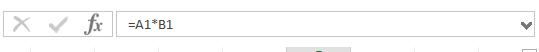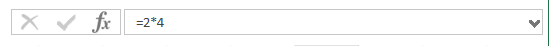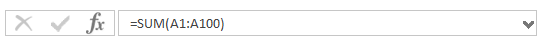# Excel Formulas Explained

Excel formulas and calculations are the basic building blocks of any Excel Workbook, and without these things any Excel workbook or worksheet is just a collection of static tabulated data that could be created in any word processor package.

A formula at its most basic basic level is a sequence of commands that that when entered in to a cell performs some calculations and returns a result which is displayed in the cell instead of the formula itself.

Formula construction

To create a formula in a cell it must first be selected as the active cell, and then in the Address bar you will need to type an = sign before starting your formula to signal to Excel that it is a formula, not a text string.A formula can come in one of three basic types

1. Mathematical Operators
2. Values or Text
3. Worksheet functions

A formula can also incorporate more than one of the three types in it’s structure

###### Mathematical Operators

Mathematical operators ( such as + – * / ) can be used to manipulate one cells contents based on that of another. e.g. – We have numbers in cells A1 and B1 ( 2 and 4 respectively) and if we select cell C1 and type the formula =A1*B1 then the contents of cell C1 will display 8. As the contents of cells are dynamic, changing the numbers in A1 or B1 will change the contents of cell C1 automatically.###### Values or Text

Numbers or formula can be entered directly in to cells and will calculate a result. e.g. if in cell C1 we type =2*4 then the cell will still display 8, however it will always display 8, no matter what else changes. For this reason constant value formula offer little to the Excel workbook developer.###### Worksheet Functions

Excel has a vast array of built in functions that will save most users a lot of time and effort. Probably the most used function is the ‘SUM’ function. Imagine a worksheet that has column A with 100 rows of numbers, that need to be totaled, you could create a formula in cell A101 that says =A1+A2+A3+A4+A5+A6+A7….. all the way up to +A99+A100 but this is obviously very tedious and time consuming, fortunately the Excel ‘Sum’ function is a lot quicker to implement. Simply type =SUM(A1:A100) into Cell A101 to achieve the same thing.Excel has over one hundred different built in functions including those concerning Date / Time, Trigonometric functions, mathematical calculations, and Lookup functions that really help to harness the power of the platform as a computational and calculation tool.